## 5.1.1 Anisotropic Distribution Function

In the current equation (2.76) a temperature tensor occurs. The diagonal components of this tensor are defined as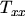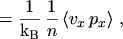(5.1)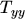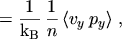(5.2)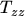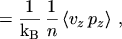(5.3)

and the temperature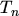used in the energy transport model is defined through the mean carrier energy (cf. eqn. (2.94))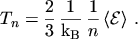(5.4)

For parabolic bands the relation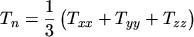(5.5)

holds.

Monte Carlo simulations of a one-dimensional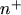-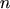-test structure show that the temperature components parallel () and normal () to the direction of the current flow are quite different (Fig. 5.4). In particular, the transverse temperature component is smaller than the longitudinal component [64, Fig.7]. This encourages the derivation of a transport model which takes an anisotropic distribution function into account.M. Gritsch: Numerical Modeling of Silicon-on-Insulator MOSFETs PDF